Courses

# NCERT Solutions - Non Competitive Markets Commerce Notes | EduRev

## Commerce : NCERT Solutions - Non Competitive Markets Commerce Notes | EduRev

The document NCERT Solutions - Non Competitive Markets Commerce Notes | EduRev is a part of the Commerce Course Economics Class 11.
All you need of Commerce at this link: Commerce

Page No 99:

Question 1: What would be shape of demand curve, so that the total revenue curve is
(i) A positively sloped straight line passing through the origin.
(ii) A horizontal line.
(a) If the total revenue curve is a positively sloped straight line passing through the origin, then the slope of the demand curve will be a horizontal line parallel to the x-axis.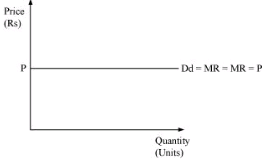(b) If the total revenue curve is a horizontal line, then the demand curve will be downward sloping.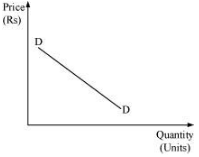Question 2: From the schedule provided below calculate the total revenue, demand curve and the price elasticity of demand.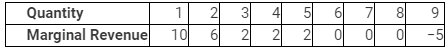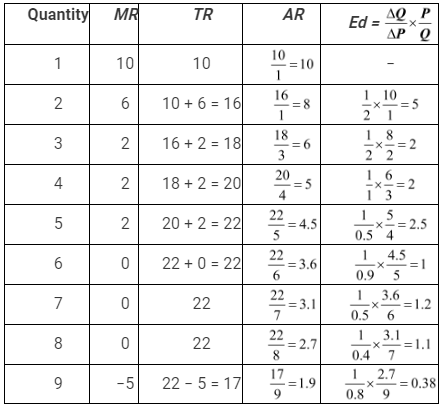Question 3: What is the value of the MR when the demand curve is elastic?
Answer: When demand curve is elastic (ed > 1), then according to the relationship MR = P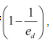the fraction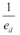will be less than 1.
Hence, MR will be positive when P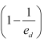is positive.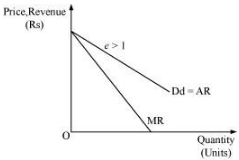Question 4: A monopoly firm has a total fixed cost of Rs 100 and has the following demand schedule: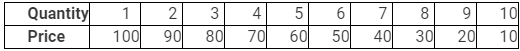Find the short run equilibrium quantity, price and total profit. What would be the equilibrium in the long run? In case the TotaI cost was Rs 1000, describe the equilibrium in the short run and in the long run.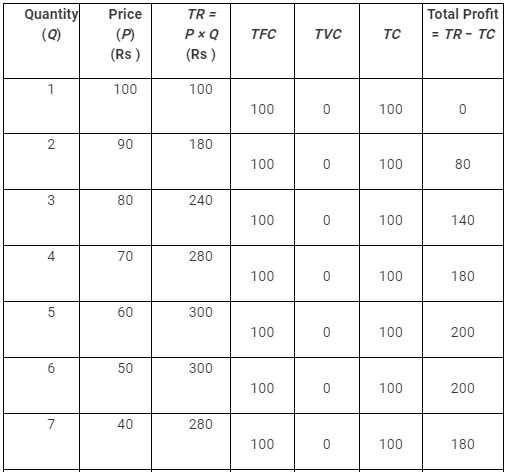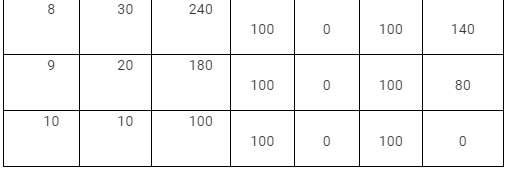Let the total variable cost of the monopolist firm is zero. Now, the profit will be the maximum where TR is maximum. That is, at the 6th unit of output the firm will be maximising its profit and the short run equilibrium price will be Rs 50.
Profit = TR − TC
= 300 − 100
Profit = Rs 200
If the total cost is Rs 1000, then the equilibrium will be at a point where the difference between TR and TC is the maximum.
TR is the maximum at the 6th level of output.
So profit = 300 − 1000
= −700
So, the firm is earning losses and not profit. As the monopolist firm is incurring losses in the short run, it will stop its production in the long run.

Page No. 100

Question 5: If the monopolist firm of Exercise 3, was a public sector firm. The government set a rule for its manager to accept the government fixed price as given (i.e. to be a price taker and therefore behave as a firm in a perfectly competitive market). And the government decide to set the price so that demand and supply in the market are equal. What would be the equilibrium price, quantity and profit in this case?
Answer: If the government sets a rule for the public sector firm to accept the fixed price, then, the monopoly firm will have to behave like a perfectly competitive firm and will be a price taker. In this case, the price fixed (Pe), as set by the government, will equate the demand and the supply, which will determine the equilibrium point ‘E’. At the price Pe, the firm earns normal profit, i.e. zero economic profit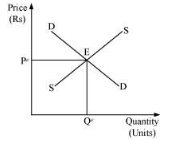Equilibrium price = Pe (fixed by the government)
Equilibrium quantity = Qe
Profit = Normal profit

Question 6: Comment on the shape of MR curve in case when TR curve is a
(i) positively sloped straight line
(ii) horizontal straight line
(i) Based on the relationship between MR and TR it can be said that when TR curve is a positively sloped straight line, then MR curve is a horizontal line. MR and demand curve are the same, and the price (AR) remains constant for different output levels. This happens under perfect competition.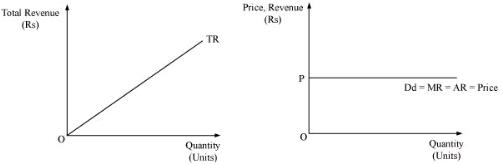(ii) When TR curve is a horizontal straight line, then MR is zero. Therefore, MR curve is also a horizontal straight line and coincides with the output-axis.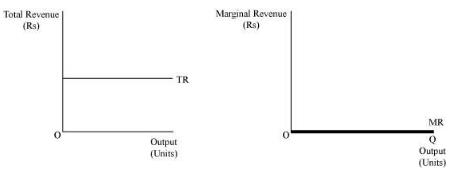Question 7: The market demand curve for a commodity and the total cost for a monopoly firm producing the commodity is given by the . schedules below.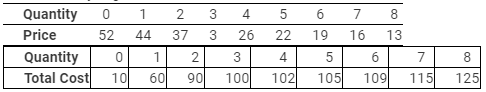Use the information to calculate the following
(a) The MR and Me schedules
(b) The quantities for which the MR and Me are equal.
(c) The equilibrium quantity of output and the equilibrium price of the commodity.
(d) The total revenue, total cost and total profit in equilibrium.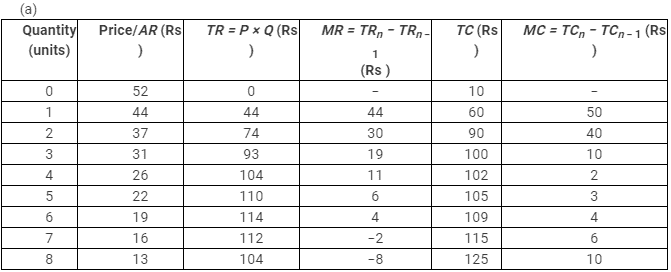(a) MR= Me at 6th unit of output
(b) Equilibrium quantity = 6 Units Equilibrium price = Rs 19
(c) At equilibrium
(d) TR = Rs 114
TC = Rs 109
Total profit = TR − TC
= Rs 114 − 109
= Rs 5

Question 8: Will the monopolist firm continue to produce in the short run if a loss is incurred at the best short run level of output?
Answer: A monopolist firm can earn losses in the short run if the price is less than the minimum of AC. But if the price falls below the minimum of AVC, then the monopolist will stop production. The firm will continue to produce when the price is in between the minimum of AVC and the minimum of AC.

Question 9: Explain why the demand curve facing a firm under monopolistic
competition is negatively sloped.

Answer: A monopolistic firm has differentiated products; thus, it has to lower its price in order to increase its sales. Further, the products of different monopolistic firms are close substitutes to each other. Hence, the demand for all the products is elastic. For this reason, the demand curve is negatively sloped.

Question 10: What is the reason for the long run equilibrium of a firm in monopolistic competition to be associated with zero profit?
Answer: The long run time horizon is featured by the free entry and exit of firms. If the firms in the short run are earning abnormal or super normal profits, then, new firms will be attracted to enter the market. Due to the new entrants, the market supply will increase. It leads to the reduction in the price that ultimately falls sufficiently to become equal to the minimum of average cost. When the market price is equal to the minimum of AC, it implies that all the firms earn normal profit or zero economic profit.
On the contrary, if in the short run the firms are earning abnormal losses, then the existing firms will stop production and exit the market. This will lead to a decrease in the market supply, which will ultimately raise the price. The price will continue to rise until it becomes equal to the minimum of AC. ‘Price = AC’ implies that in the long run all the firms will earn zero economic profit.
Hence, when the price is equal to the minimum of AC, neither any existing firm will exit nor any new firm will enter the market.

Question 11: List the three different ways in which oligopoly firms may behave.
Answer: Oligopoly firms may behave in the following three ways:
1) Cartel − In order to avoid undue competition, oligopolistic firms may engage in formal agreements or contracts. This will not only allow them to maximise their total profits together, but also capture a significant market portion.
2) Informal understanding − Each firm may decide on its own, how much units of output is to be produced for maximising its individual profit, assuming that other firms would not change their strategies and decisions regarding the units of output to be produced.
3) Advertisement and differentiated product − It may happen that the firms realise that price competition will leave them nowhere and consequently they emphasise more on advertising their products. It will enable them to capture the minds of consumers and indirectly increase their market portion.

Question 12: If duopoly behavior is one that is described by Cournot, the market demand curve is given by the equation q = 200 – 4p and both the firms have zero costs, firms the quantity supplied by each firm in equilibrium and the equilibrium market price.
Q = 200 − 4p
When the demand curve is a straight line and total cost is zero, the duopolist finds it most profitable to supply half of the maximum demand of a good.
At P = Rs 0, market demand is
Q = 200 − 4 (0)
= 200 units
If firm B does not produce anything, then the market demand faced by firm A is 200 units.
∴ The supply of firm A =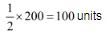In the next round, the portion of market demand faced by firm B is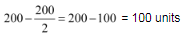∴ Firm B would supply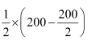= 50 units
Thus, firm B has changed its supply from zero to 50 units. To this firm A would react
accordingly and the demand faced by firm A will be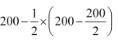= 200 − 50
= 150 units
Firm A would supply =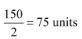The quantity supplied by firm A and firm B is represented in the table below.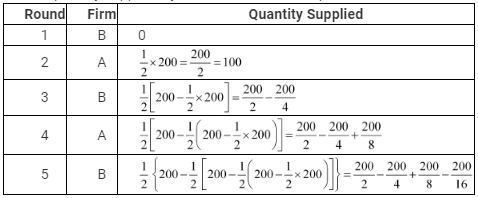Therefore, the equilibrium output supplied by firm A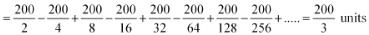Similarly, the equilibrium output supplied by firm B =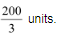Market Supply = Supply by firm A + Supply by firm B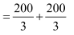Equilibrium output or Market Supply = Q =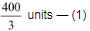For equilibrium price
Q = 200 − 4p
4p = 200 − Q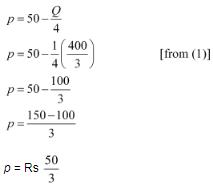Therefore, the equilibrium output (total) is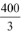units and equilibrium price is Rs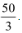Question 13: What is meant by prices being rigid? How can oligopoly behavior lead to such an outcome?
Answer: Price rigidity implies that the price is unresponsive to the changes in demand. This is because of the fact that even if any firm raises the price of its product with the motive of earning higher profits, the other firm will not do so, and the first firm will lose its customers. On the other hand, if one firm lowers its price in order to earn higher profits by maximising its sales, then in response, the other firm may also reduce the price. Consequently, the increase in total market sales is shared by both the firms. The firm that initiated selling at a lower price may get a lower share of the increase than expected.
Therefore, the firms do not change their prices due to the fear of rival’s reaction. Hence, there is no incentive for any firm to change its price. That is why the prices are regarded as rigid prices or sticky prices.

Offer running on EduRev: Apply code STAYHOME200 to get INR 200 off on our premium plan EduRev Infinity!

## Economics Class 11

213 videos|199 docs|48 tests

,

,

,

,

,

,

,

,

,

,

,

,

,

,

,

,

,

,

,

,

,

;